Function Repository Resource:

# PlouffeP

Get the value of the Plouffe P function

Contributed by: Wolfram Staff
 ResourceFunction["PlouffeP"][s,b,m,{a1,a2,…}] gives the value the value of the Plouffe P function for integers s,b,m and {a1,a2,…,am}. ResourceFunction["PlouffeP"][s,b,A] gives ResourceFunction["PlouffeP"][s,b,Length[A],A] for the List A.

## Details and Options

ResourceFunction["PlouffeP"] gives the value of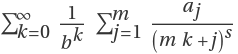, where s and b are integers and {a1,a2,,am} is a List of m integers.

## Examples

### Basic Examples (2)

The Plouffe P function is a compact way to represent a class of infinite series that sum to well-known numbers:

 In:=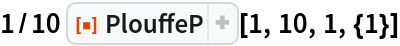Out=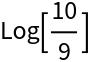Use the three-argument form:

 In:=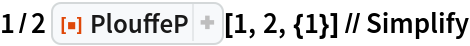Out=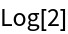### Neat Examples (1)

This example is the famous Bailey-Borwein-Plouffe formula (BBP formula):

 In:=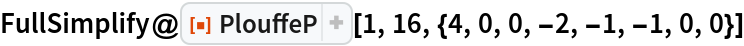Out=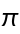George Beck

## Version History

• 1.0.0 – 13 February 2020